# A Short History of Quantum Mechanics

by James V Stone, University of Sheffield, UK.

Max Planck, a 42-year-old pillar of the scientific elite, had no intention of killing off classical physics. Despite this, he effectively hammered the first nail into the coffin of classical physics in 1900, and by 1926 it was under six feet of earth, with a headstone that could have said, “Nice Try”.

By 1900, it was known that an oven at a given temperature emits radiation, and that the spectrum of radiation has the same characteristic form irrespective of the shape of the oven. These facts suggested there was something fundamental about these blackbody spectra, shown in Figure 1. However, before 1900, no equation had been discovered that would fit blackbody spectra, because the physics underpinning such spectra was not understood.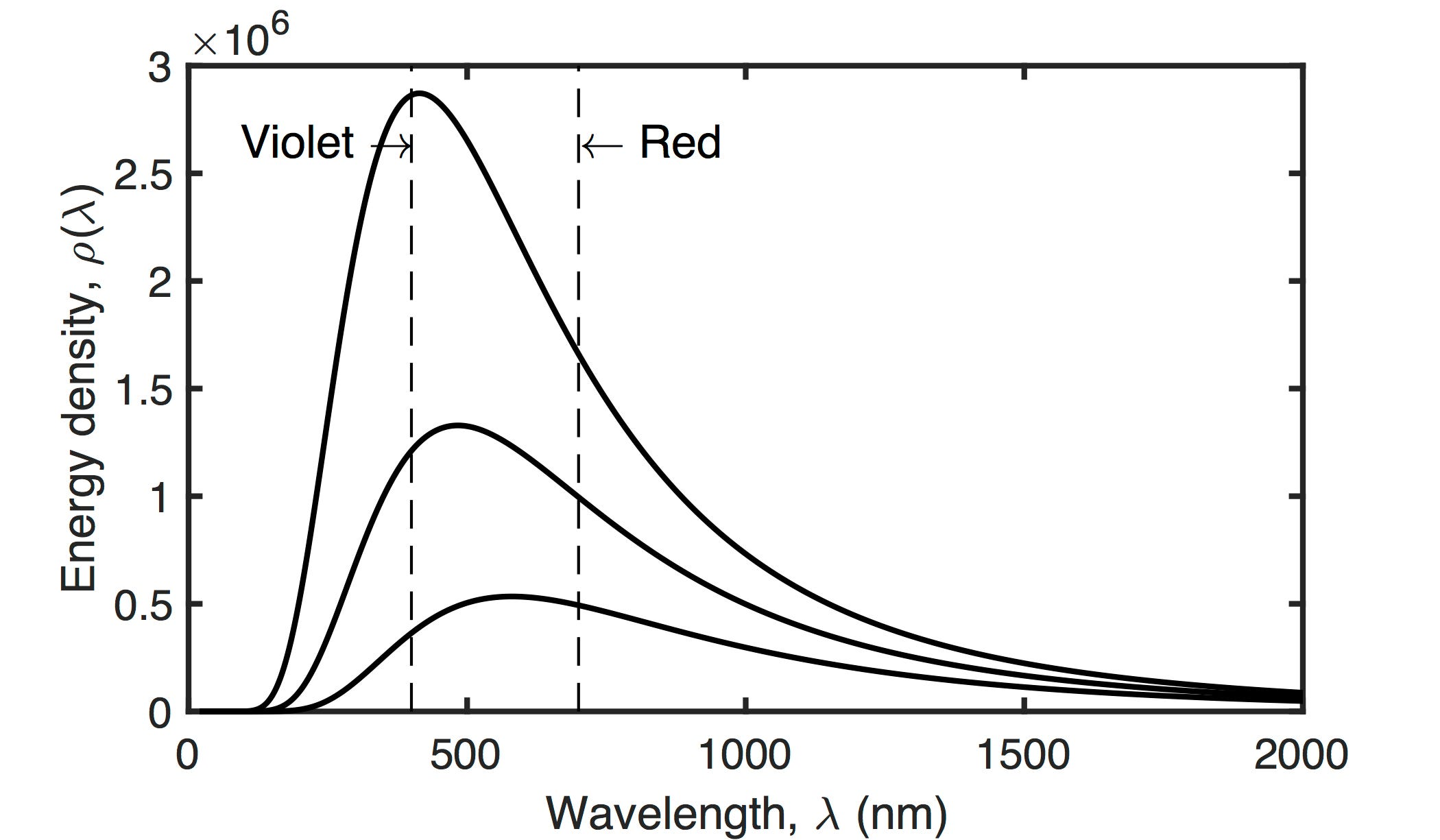Figure 1: Blackbody spectra at 7000 K (top curve), 6000 K (middle curve) and 5000 K (bottom curve). The dashed vertical lines correspond to the wavelengths of violet and red light.

In historical terms, the problem of blackbody radiation was not considered hugely important; it was just one of a handful of unremarkable problems waiting to be solved. Given the successful track record of 19th-century classical physics, it seemed reasonable to assume that the problem of blackbody radiation would also be solved before long. And so it was, but at a price.

By harnessing the tools introduced in Boltzmann’s statistical mechanics, Planck was able to fit a function to the blackbody spectra. However, the fit could be made exact only if Planck, in his self-proclaimed act of desperation, was willing to sacrifice a central pillar of classical physics, namely that the energy of electromagnetic radiation can always be absorbed or emitted by matter in any continuous amount. In its place, Planck assumed that electromagnetic waves are absorbed or emitted only at discrete energy values. Specifically, he assumed that the energy of a wave with frequency ν can change only in quanta of size , where h is Planck’s constant. Planck was confident that his ‘quantum trick’ would soon be replaced by a more traditional, classical solution. But within five years, the young Einstein had harnessed Planck’s quantum trick to throw the first handful of earth onto the coffin of classical physics.

It had been known for a long time that shining a light on metal somehow allows electric charge to flow away from the metal. And since the discovery of electrons by JJ Thomson in 1897, it was also known that this charge is carried by electrons, as if the light ejected electrons from the metal surface. In support of the idea that charge is carried by electrons, it was found that the amount of charge flowing away from an illuminated metal surface increases with the light intensity. However, it was also found that no electrons are ejected if the frequency of light falls below a critical value, even if the light is extremely intense; this is the photoelectric effect. If, for example, radiation simply shook atoms in the metal until some electrons fell out then the amount of charge released should not depend on the frequency of light. But Einstein had read Planck’s work, and the effect on him was profound:

as if the ground had been pulled from under one, with no firm foundation to be seen anywhere.

In fact, the effect on Einstein was so profound that he proposed that not only is light absorbed or emitted by matter at discrete energy values or quanta, but light at a given frequency can only exist at discrete energy values. Einstein’s photoelectric equation required the existence of packets of light, which eventually came to be known as photons. By 1909, Einstein had correctly predicted a duality between particles and waves, and that

The next phase of development in theoretical physics will bring us a theory of light that can be interpreted as a kind of fusion of the wave and particle theories.

Despite a decade of resistance (from his fellow physicists) to the idea that light is quantised into photons, Einstein was awarded the Nobel prize for this work.

All of this was set against the backdrop of a physics community in search of the essence of matter and energy. At that time, positive and negative charges were thought to be distributed evenly throughout matter, which is why this was known as the plum pudding model.

Then, in 1911, Ernest Rutherford fired alpha particles, known to be positively charged, at a thin sheet of gold foil. Most of the particles passed straight through the foil, but some were deflected to one side, and a few even reversed direction. In order to explain this, Rutherford postulated that the deflected alpha particles must be hitting something pretty solid, and he proposed that those somethings must be places where the positive charges of matter were concentrated. The result was Rutherford’s solar system model of the atom, in which many negatively charged electrons orbit each positively charged nucleus, like planets around the sun (see Figure 2).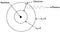Figure 2: Rutherford’s solar system model of the atom. When an electron falls from the orbit with principal quantum number n2 = 3 to the one with n2 = 2, the energy ∆E released creates a photon with frequency ν = ∆E/h, where h is Planck’s constant.

But there was a problem: given that the nucleus is positively charged and that each electron is negatively charged, every electron ought to spiral down towards the nucleus. To salvage Rutherford’s solar system atomic model, in 1913 Niels Bohr proposed that electrons can exist only in fixed orbits, which he called stationary states.

Of course, Bohr knew of Planck’s and Einstein’s work on quantum energy, so it was natural to suppose that these stationary states were related to Planck’s constant. Specifically, Bohr proposed that electron orbits have fixed, quantised angular momenta which exist only in multiples of h/() (the angular momentum of an electron with mass M travelling at speed v around a circular orbit of radius r is Mvr). As a result, quantised angular momenta implied corresponding quantised energy levels for electrons. In support of his model, Bohr confirmed the Balmer formula for the emission spectra of hydrogen atoms (Figure 3), which had hitherto been a purely empirical formula devised by Johann Balmer in 1885. Despite this success, Bohr’s atom was considered a flawed mixture of classical and quantum ideas. Even so, his work acted as a spur to find a more complete quantum theory. In this way, Bohr contributed his own handful of earth to the final resting place of classical physics.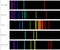Figure 3: Emission spectra of different elements, with wavelength increasing from left to right. Each set of emission lines is obtained by heating an element as a gas.

Over the next decade, the key ideas of Planck, Einstein and Bohr permeated the physics community. As is often the case, ideas that seemed barely credible to established physicists appeared quite natural to the newly minted physicists of the next generation. So it was that the young Prince Louis de Broglie proposed in 1924 that, just as light waves can behave as if they are particles, particles can behave as if they are waves, which exist only in discrete quanta. As de Broglie said:

When I conceived the first basic ideas of wave mechanics in 1923–24, I was guided by the aim to perform a real physical synthesis, valid for all particles, of the coexistence of the wave and of the corpuscular aspects that Einstein had introduced for photons in his theory of light quanta.

But de Broglie did not just make a bold claim — he also quantified that claim with an equation. Briefly, de Broglie’s equation for a particle with momentum p is p = h/λ, where h is Planck’s constant and λ is the wavelength (Figure 4). As de Broglie intended, his equation provided a quantum mechanical justification for Bohr’s model.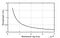Figure 4: The relationship between momentum and de Broglie wavelength for an electron.

With the advent of de Broglie’s proposal, it became possible to explain the form of Bohr’s stationary orbits in terms of de Broglie wavelengths of electrons. In essence, Bohr’s quantisation rule for angular momentum is equivalent to insisting that the orbit of each electron has a circumference that is equal to an integer number of de Broglie electron wavelengths (Figure 5).

For an electron with mass M travelling at velocity v, its de Broglie wavelength is λ = h/(M v). If only an integer number n of de Broglie wavelengths λ are permitted in a circular orbit with radius r then the orbital circumference is 2πr = λ = hn /(M v), and therefore r = hn /(2πMv). For n=1, this correctly predicts the value of the Bohr radius of a hydrogen atom as a0=0.0529nm.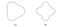Figure 5: Electron waves with integer numbers n of de Broglie wavelengths in imaginary two-dimensional atoms: (a) n = 3; (b) n = 4.

Of course, an equation is of little use if it cannot be tested by experiment. In this case, de Broglie’s equation predicted that electrons should diffract and interfere with each other to produce interference patterns similar to those observed in (light) double-slit experiments (Figures 6&7).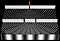Figure 6: The double-slit experiment. Waves travel from the source (top) until they reach the first barrier, which contains a slit. A semi- circular wave emanates from the slit until it reaches the second barrier, which contains two slits. The two semi-circular waves emanating from these slits interfere with each other, producing peaks and troughs along radial lines that form an interference pattern on a screen (bottom).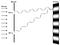Figure 7: Waves travel from the left until they hit the barrier, which contains two slits, allowing a wave to emerge from each slit. These two waves interfere with each other and form an interference pattern on a screen (right). The waves shown are out of phase at a point on the screen, so they interfere destructively.

Within three years that prediction had been verified (Figure 8), so there could be no doubt that matter, in the form of electrons, behaves as if it were being guided by what de Broglie called a pilot wave. This finally put to rest any argument regarding the relationship between light, energy and matter. In effect, de Broglie defined the universe in terms of just two natural kinds, waves and matter, with energy acting as the currency for converting between them. Not only was matter now considered to be a wave, but that wave was quantised by the same constant used to quantise light. Like Planck, Einstein and Bohr, de Broglie had cast his own handful of earth into the grave of classical physics.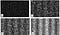Figure 8: The gradual emergence of an interference pattern in a double- slit experiment. Each dot represents the position of one electron, and the temporal sequence is b, c, d and then e. Reproduced with permission from Dr Tonomura’s Wikimedia image.

The search for a coherent quantum theory was almost over. In 1925, the German wunderkind Werner Heisenberg effectively reinvented matrix algebra in the process of constructing matrix mechanics. At that time, matrix algebra was not familiar to most physicists, which is probably why Heisenberg unwittingly reinvented it, and why his matrix mechanics was not widely adopted. In contrast, physicists were familiar with Maxwell’s equations for electromagnetic waves, which may be why they found Erwin Schrödinger’s wave mechanics much easier to digest.

In 1926, Schrödinger (acting on a suggestion by Debye) reasoned that if there is a de Broglie wave then there should be a wave equation to go with it, and the result is now known as the Schrödinger equation. This equation cannot be derived from classical physics, nor using any conventional process of deduction; it is essentially a product of Schrödinger’s intellect. Later that year, Schrödinger showed that his wave mechanics and Heisenberg’s matrix mechanics are equivalent.

In that remarkable year, Paul Dirac, having read a draft of Heisenberg’s thesis in 1925, devised yet another framework for quantum mechanics, called transformation theory. Happily, Dirac (with Jordan) proved that Heisenberg’s matrix mechanics and Schrödinger’s wave mechanics are special cases of Dirac’s transformation theory. Thus, by the end of 1926, there were three different, but equivalent, formulations of quantum mechanics. Three more handfuls of earth had now rendered classical physics almost invisible.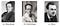Figure 9: The authors of three equivalent theories of quantum mechanics.

However, all three formulations suffered from an apparent anomaly, namely their prediction that if the momentum of a particle could be measured exactly then its position could be anywhere in the universe, and if the position of a particle could be measured exactly then its momentum could have any value. More precisely, all three formulations predicted that the product of the uncertainties in momentum and position cannot be less than a critical value, so if one is made smaller then the other must be made larger. This is the famous Heisenberg uncertainty principle, discovered in 1927.

Of course, no matter how elegant a theory is, and no matter how many physicists have developed equivalent formulations of that theory, it must eventually make contact with the everyday world of experience. Even though quantum mechanics was supremely good at predicting experimental results at the microscopic scale of particles, those predictions should always be compatible with experimental results at the macroscopic scale of everyday objects. This general idea was formalised by Bohr in 1923 as the correspondence principle, which states that the predictions of quantum mechanics must become indistinguishable from the predictions of classical physics at macroscopic scales. In other words, the microscopic physics of quantum mechanics must be consistent with the macroscopic world of classical physics.

Despite the enormous progress that had been made up to 1927, this was by no means the end of the story of quantum mechanics. In the years that followed, lively debates raged over the interpretation of quantum mechanics. Of these debates, one has a special status, not only because it took about thirty years to resolve, but because it represented the final handful of soil cast into the neglected grave of classical physics.

After several false starts, Albert Einstein and his colleagues Boris Podolsky and Nathan Rosen finally came up with a watertight proof that the predictions of quantum mechanics were incompatible with reality; more specifically, the predictions were incompatible with Einstein’s own theory of special relativity, published in 1905. In essence, the famous EPR paper, published in 1935, predicted that two particles could be created in such a way that they share the same Schrödinger wavefunction, so that they are entangled. This means that if the state of one of the particles is measured then the other particle instantly adopts a state compatible with that of its partner, as if the particles can communicate with each other. Such instantaneous action can occur only if the particles communicate with each other at a speed that is effectively infinite. However, the instantaneous communication predicted by the EPR paper is incompatible with special relativity, which states that faster-than-light communication is impossible. The problem was that, for almost thirty years, no-one could come up with an experiment that could test the predictions of the EPR paper.

Then, in 1964, John Bell published his ingenious theorem (now known as Bell’s theorem), which showed how the idea of the EPR paper could be tested. Over the subsequent years, increasingly accurate experiments were carried out, and the increasingly convincing results yielded the same conclusion. Today, it is generally accepted that measuring the state of one particle not only determines the state of that particle but also determines the state of its entangled partner, as if they communicate with each other instantly. Ironically, the EPR paper, which began life as a thought experiment designed to undermine the validity of quantum mechanics ultimately provided the means (in the form of Bell’s theorem) to remove all possible doubt of its truth.

# Summary

1900 Planck proposes that light interacts with matter in discrete quanta.

1905 Einstein proposes that light exists only as quanta, which came to be known as photons.

1913 Bohr announces the solar system model of electron orbits and applies Planck’s quantization idea to angular momentum, thus explaining the emission lines of the hydrogen spectrum.

1924 de Broglie proposes that matter behaves like a wave, and provides an equation relating momentum to the wavelength of matter waves.

1925 Heisenberg develops matrix mechanics in his doctoral thesis and publishes the first paper on quantum mechanics. Schrödinger uses de Broglie’s matter waves to develop wave mechanics, published in 1926.

1926 Dirac proves that the models of Heisenberg and Schrödinger and his own transformation mechanics are equivalent.

1927 Heisenberg’s uncertainty principle is published. The existence of matter waves is confirmed for electrons.

1935 The EPR paper claims that quantum mechanics predicts particle entanglement, which implies faster-than-light communication, contradicting Einstein’s special theory of relativity from 1905.

1935 Schrödinger’s cat is born.

1964 Bell’s theorem provides a recipe for testing the particle entanglement predicted by the EPR paper.

1972 Clauser provides the first experimental evidence that Bell’s inequality is violated and, therefore, that Einstein’s ‘spooky action at distance’ exists13.

1976 Ingarden publishes the paper “Quantum Information Theory”.

1982 Aspect confirms Clauser’s result and closes the locality loophole.

1985 Deutsch describes the first universal quantum computer.

1994 Shor invents the first quantum computer algorithm.

2000 McFadden publishes the book Quantum Evolution.

2004 Quantum-state teleportation between calcium ions is demonstrated.

Note: This is an edited extract from The Quantum Menagerie by James V Stone.